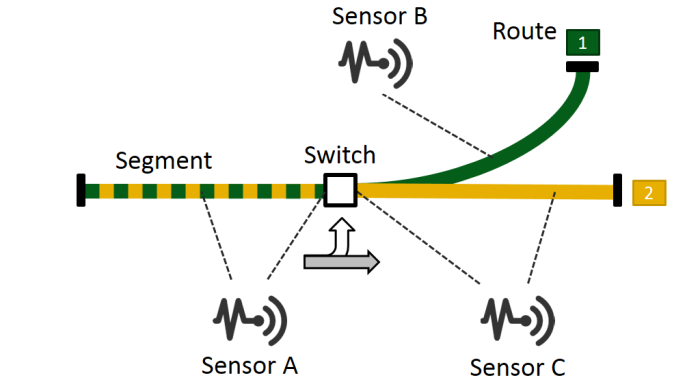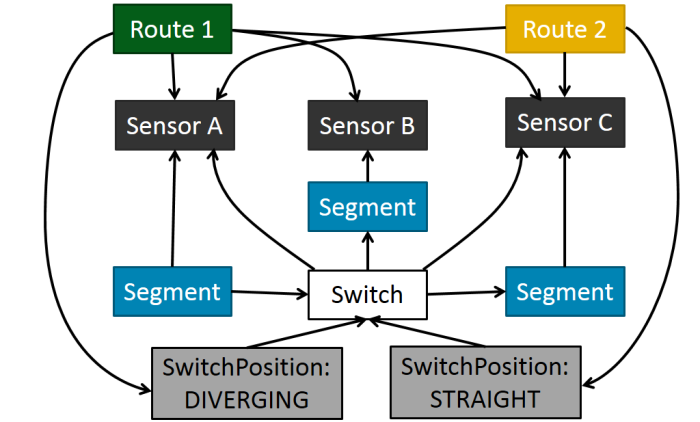# Multidimensional graph metrics with Neo4j and Cypher

The goal of this Gist is to demonstrate the graph metrics collected in our paper Towards the characterization of realistic models: evaluation of multidisciplinary graph metrics.

## Dataset

As an example, we use a simple railway network consisting of two Routes, three Segments, a Switch and three Sensors.Figure 1. Railway network

A possible graph representation of the example as a graph is this:Figure 2. Railway graph

This short video demonstrates how the railway network is transformed to a graph.

The following query creates the graph (the query is automatically executed when loading the page).

In the following, we present the formal definitions of the metrics and evaluate them on the example graph using Cypher queries. For the details of the notation, see the paper.

## One-dimensional metrics

### Clustering coefficient

$$C(v) = \frac {\big| a, b \mid \mathit{Connected}(v, a) \land \mathit{Connected}(v, b) \land \mathit{Connected}(a, b) \big|} {\big| a, b \mid \mathit{Connected}(v, a) \land \mathit{Connected}(v, b) \big|}$$

## Multidimensional metrics

### Metrics interpreted on dimension-node pairs

#### Dimensional degree

$$\mathit{Degree}\left(v,d\right) = \left|\big\{ w \in V \mid \mathit{Connected}(v, w, d) \big\}\right|$$

### Metrics interpreted on dimensions

#### Node dimension activity

$$\mathit{NDA}(d) = \big| \{ v \in V \mid \mathit{Active}(v,d) \} \big|$$

#### Node dimension connectivity

$$\mathit{NDC}(d) = \frac{\mathit{NDA}(d)}{|V|}$$

#### Edge dimension activity

$$\mathit{EDA}(d) = \big| \{ (v,w,d) \in E \mid v,w \in V \} \big|$$

#### Edge dimension connectivity

$$\mathit{EDC}(d) = \frac{\mathit{EDA}(d)}{|E|}$$

### Metrics interpreted on nodes

#### Node activity

$$\mathit{NA}(v) = \big| \{ d \in D \mid \mathit{Active}(v, d) \} \big|$$

#### Multiplex participation coefficient

$$\mathit{MPC}(v) = \frac{|D|}{|D| - 1} \left[ 1 - \sum\limits_{d\in D}^{}{\left(\frac{\mathit{Degree}(v,d)}{\mathit{Degree}(v,D)}\right)^2} \right]$$
##### Dimensional clustering coefficients

DC1 variant

$$\mathit{DC}_1(v) = \frac {\big| a, b \mid \mathit{Connected}(v, a, d_1) \land \mathit{Connected}(v, b, d_1) \land \mathit{Connected}(a, b, d_2) \land d_1 \neq d_2 \big|} {\big| a, b \mid \mathit{Connected}(v, a, d_1) \land \mathit{Connected}(v, b, d_1) \big|}$$

DC2 variant

$$\mathit{DC}_2(v) = \frac {\big| a, b \mid \mathit{Connected}(v, a, d_1) \land \mathit{Connected}(v, b, d_2) \land \mathit{Connected}(a, b, d_3) \land d_1 \neq d_2 \land d_2 \neq d_3 \land d_1 \neq d_3 \big|} {\big| a, b \mid \mathit{Connected}(v, a, d_1) \land \mathit{Connected}(v, b, d_2) \land d_1 \neq d_2 \big|)}$$

#### Metrics interpreted on dimension pairs

The node activity binary vector for each node v is defined as:

$$a_v = \left\{ a_v^{},a_v^{}, \dots, a_v^{[|D|]} \right\}, \text{where } a_v^{[d]} = \begin{cases} 1, & \text{if } \mathit{Active}(v,d),\\ 0, & \text{otherwise} \end{cases}$$

Using this vector, the pairwise multiplexity metric is:

$$Q(d_1, d_2) = \frac{1}{|V|} \sum_{v \in V} {a_v^{[d_1]} a_v^{[d_2]}}$$